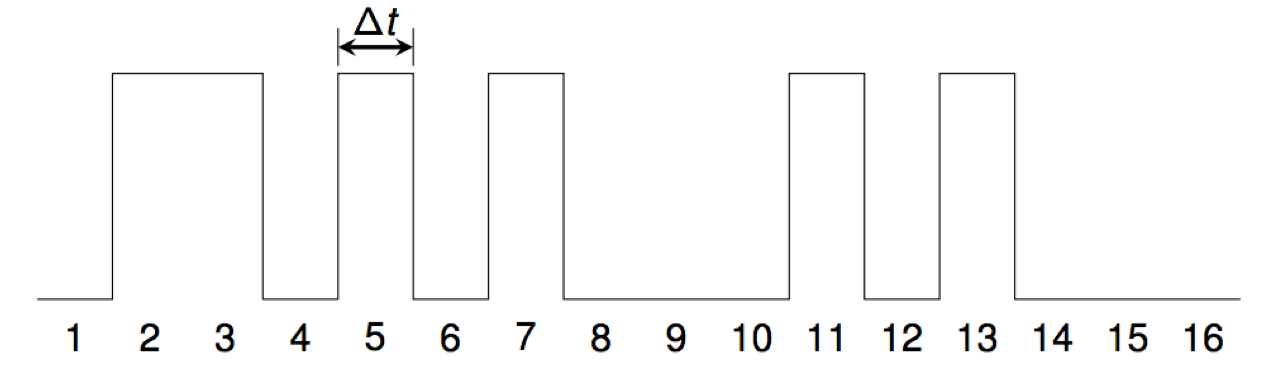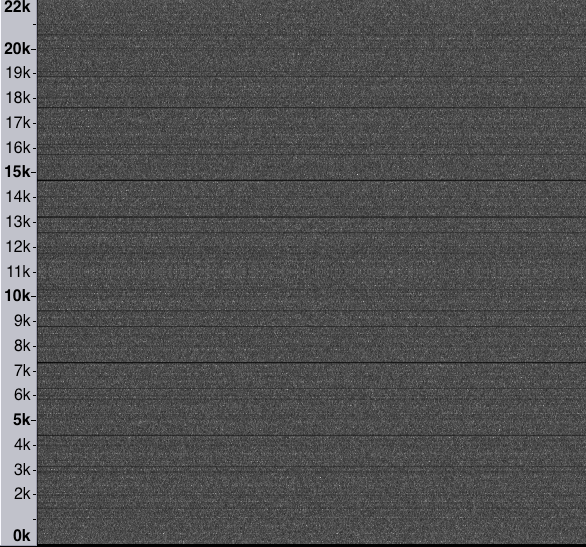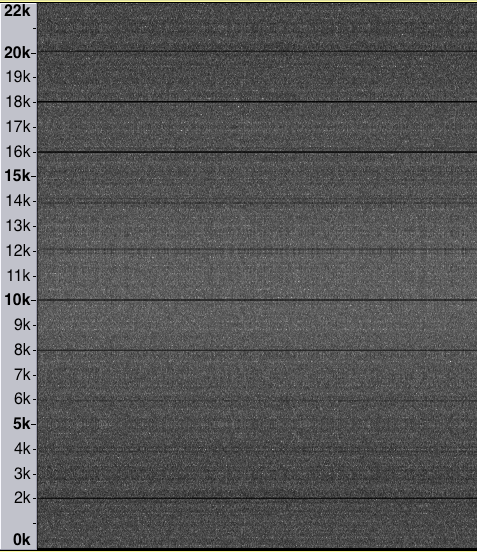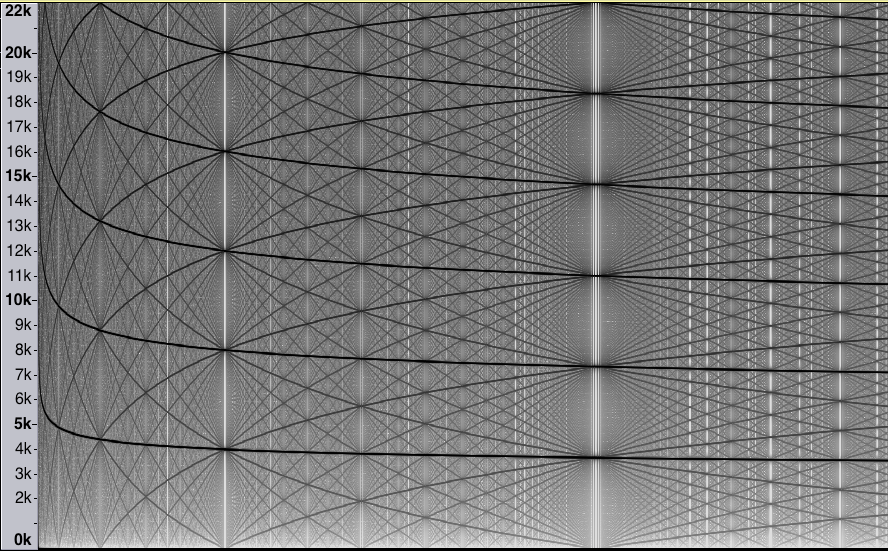This is the home page for the WXML project Number Theory and Noise. The project was started in Spring 2016 by Matthew Conroy and is ongoing [but is on hiatus durind the pandemic]. In this project, students investigate the possibilities arising from the representations of certain integer sequences (specifically, increasing sequences of positive integers) as sounds.

# Project Intro

A digital audio file is created from a given set A of positive integers by setting sample number i to a non-zero constant c for all i in the set A. All other samples are set to zero.

For example, the waveform for the primes starts like this:We use the standard CD-audio sampling rate of 44100 samples per second, so Δt = 1/44100= 0.0000226757... seconds. By using a fixed sampling rate, the sounds are comparable, one to the other.

For many sets, the result is what most people would describe as noise, though there are many, many variations.

Below are a few examples to illustrate what the project is about.

## The primes

Below you can hear the sound generated from the prime numbers less than 106. This is quite noisy sound, but it is not white noise: certain frequencies are emphasized over others. The "white noise" sound below is generated from a randomly generated sequence of positive integers with the same overall density as the primes to 106.

Here is a spectrogram of the primes sound. The black horizontal lines indicate frequencies that are emphasized in the sound. Many correspond to frequencies of the form 44100/n, where n is a small positive integer, and integer multiples of these frequencies.It is worth noting that the complement of a sequence of positive integers (among the positive integers) will generate a sound identical to the original sequence: the waveform is simply negated, so it sounds exactly the same. The spectrogram above can be thought of as the spectrogram of the composite numbers, too.

## The Ulam numbers

The Ulam numbers are sequence of positive integers created by the following rule. The first two terms are 1 and 2. We then add to the sequence the smallest positive integer not yet in the sequence which can be represented as a sum of earlier terms in exactly one way. So the next terms are 3 and 4. Since 5 can be written as 1+4 or 3+2, it does not get added, so the next term is 6.

The sequence begins 1, 2, 3, 4, 6, 8, 11, 13, 16, 18, 26, 28, 36, 38, 47, 48,... .

This sequence has a very complex structure and not much has been proved about it.

Here is the sound.

Here is the spectrogram of this sound.Several prominent spectral lines can be seen in the spectrogram. One is at about 2040 hz. This corresponds to a known clustering of the Ulam sequence with period about 21.6. Another is seen at about 18040 hz. This corresponds to the phenomenon described by Stefan Steinerberger in his paper, A Hidden Signal in the Ulam Sequence.

## Floor of n log n

This sequence demonstrates a bit of the variety of the sounds found in this project: they are not all equally noisy, or noisy in the same way.

Here is the sound.

 OIES id description sound player creator A050504 floor of n log n download Hannah Claire Van Wyk

And here is the spectrogram of the sound.